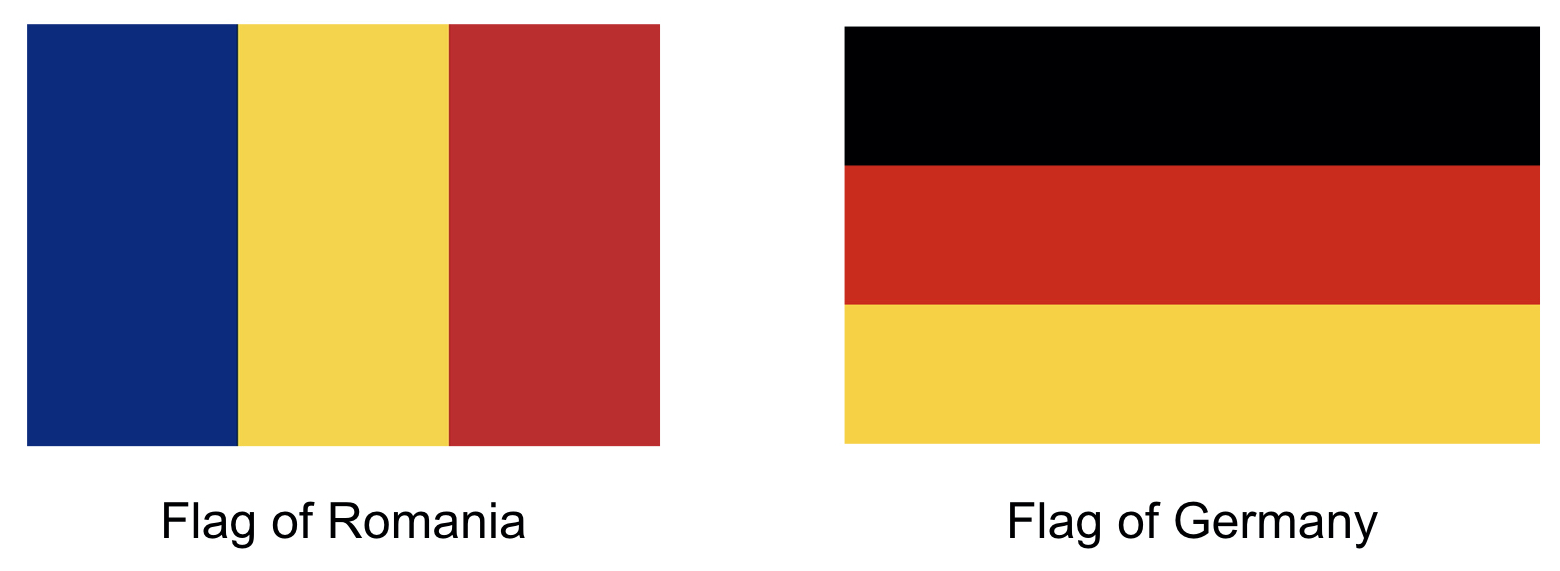# Less is More

Recall that, in Problem Set 6, you wrote code to analyze sequences of DNA. Those sequences of DNA were stored in text files that might resemble the below.

``````AAAAAAATTTTTAAAGGGGCCCCCCAAACCCC
``````

Some of those text files, though, were quite large. And we gave you quite a few such files!

It turns out we could have compressed those files by representing those sequences differently. Rather than store one character per nucleotide (`A`, `C`, `G`, or `T`), we could have stored one character (plus a number) per “run” of nucleotides, whereby a run is a sequence of identical values. For instance,

• `A` could instead be encoded as `A1`,
• `TTT` could instead be encoded as `T3`, and
• `CCCCCCCCCC` could instead be encoded as `C10`.

This type of compression is known as “run-length encoding,” wherein runs of values are encoded by storing the number of repetitions.

Our original sequence of 32 nucleotides, `AAAAAAATTTTTAAAGGGGCCCCCCAAACCCC`, could thus be encoded `A7T5A3G4C6A3C4` with only 14 characters, thereby decreasing the length of our sequence (and number of bytes) by more than half!

1. (1 point.) Consider the DNA sequence below.

`````` TTTAAAACCGAAA
``````

How could that sequence instead be encoded using run-length encoding?

While run-length encoding will result in smaller file sizes for some DNA sequences, for other sequences this algorithm might actually increase the size of the file.

1. (2 points.) For what types of sequences would this algorithm likely increase the file size, rather than decrease it? Include in your answer an example of a DNA sequence for which the “compressed” version actually requires more characters than the original.

It turns out that run-length encoding can be used to compress images as well. Recall that (24-bit) BMP files can be thought of as a sequence of rows, where each row consists of pixels, and each pixel is represented using 1 byte for an amount of red, 1 byte for an amount of green, and 1 byte for an amount of blue.

Consider what might happen if, instead of storing every pixel, we were to compress images using run-length encoding, where runs of the same pixel within a row were stored as a pixel, followed by a count.

1. (2 points.) Imagine what might happen if we compressed BMPs of the flags of Romania and Germany, below, and compressed each using run-length encoding for each row of pixels. Assuming both flags have the same resolution (i.e., rows and columns of pixels), which image could be compressed more (i.e., take up less space once compressed)? Why?Let’s now revisit our encoding of DNA and consider a different method altogether for storing a DNA sequence using fewer bytes. Recall that Problem Set 6’s files were stored using ASCII, where each character in the text file took up 1 byte (8 bits) of space. According to ASCII,

• `A` is represented as `01000001` (which is 65 in decimal),
• `C` is represented as `01000011` (which is 67 in decimal),
• `G` is represented as `01000111` (which is 71 in decimal), and
• `T` is represented as `01010100` (which is 84 in decimal).

An ASCII encoding is useful if our text files will contain many different characters: uppercase letters, lowercase letters, numbers, punctuation, and/or the like. But if the only characters we need our file to store are `A`, `C`, `G`, and `T`, then we could use fewer bits to represent each nucleotide.

• `A` is represented as `0`
• `C` is represented as `1`
• `G` is represented as `10`
• `T` is represented as `11`
Thus, the sequence `AGCC` would be represented as `01011`. Only 5 bits, instead of the original 32!
1. (1 point.) Using this new encoding, how would you represent the sequence `CCCAGTTA` in binary?
2. (1 point.) Using this new encoding, how would you represent the sequence `TGCATCCA` in binary?